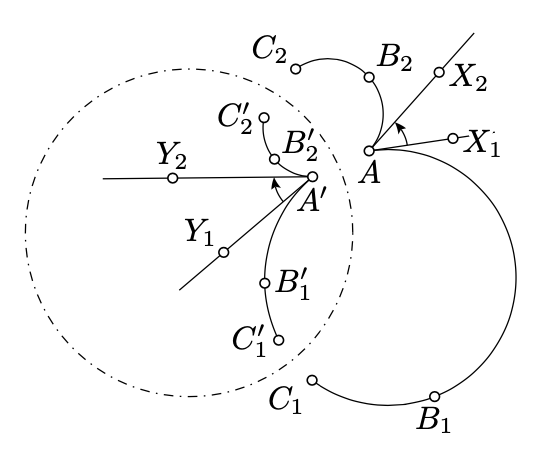$$\newcommand{\id}{\mathrm{id}}$$ $$\newcommand{\Span}{\mathrm{span}}$$ $$\newcommand{\kernel}{\mathrm{null}\,}$$ $$\newcommand{\range}{\mathrm{range}\,}$$ $$\newcommand{\RealPart}{\mathrm{Re}}$$ $$\newcommand{\ImaginaryPart}{\mathrm{Im}}$$ $$\newcommand{\Argument}{\mathrm{Arg}}$$ $$\newcommand{\norm}{\| #1 \|}$$ $$\newcommand{\inner}{\langle #1, #2 \rangle}$$ $$\newcommand{\Span}{\mathrm{span}}$$

# 10.6: Angles after inversion

$$\newcommand{\vecs}{\overset { \rightharpoonup} {\mathbf{#1}} }$$ $$\newcommand{\vecd}{\overset{-\!-\!\rightharpoonup}{\vphantom{a}\smash {#1}}}$$$$\newcommand{\id}{\mathrm{id}}$$ $$\newcommand{\Span}{\mathrm{span}}$$ $$\newcommand{\kernel}{\mathrm{null}\,}$$ $$\newcommand{\range}{\mathrm{range}\,}$$ $$\newcommand{\RealPart}{\mathrm{Re}}$$ $$\newcommand{\ImaginaryPart}{\mathrm{Im}}$$ $$\newcommand{\Argument}{\mathrm{Arg}}$$ $$\newcommand{\norm}{\| #1 \|}$$ $$\newcommand{\inner}{\langle #1, #2 \rangle}$$ $$\newcommand{\Span}{\mathrm{span}}$$ $$\newcommand{\id}{\mathrm{id}}$$ $$\newcommand{\Span}{\mathrm{span}}$$ $$\newcommand{\kernel}{\mathrm{null}\,}$$ $$\newcommand{\range}{\mathrm{range}\,}$$ $$\newcommand{\RealPart}{\mathrm{Re}}$$ $$\newcommand{\ImaginaryPart}{\mathrm{Im}}$$ $$\newcommand{\Argument}{\mathrm{Arg}}$$ $$\newcommand{\norm}{\| #1 \|}$$ $$\newcommand{\inner}{\langle #1, #2 \rangle}$$ $$\newcommand{\Span}{\mathrm{span}}$$

Proposition $$\PageIndex{1}$$

In the inversive plane, the inverse of an arc is an arc.

Proof

Consider four distinct points $$A, B, C$$, and $$D$$; let $$A', B', C'$$, and $$D'$$ be their inverses. We need to show that $$D$$ lies on the arc $$ABC$$ if and only if $$D'$$ lies on the arc $$A'B'C'$$. According to Proposition 9.5.1, the latter is equivalent to the following:

$$\measuredangle ADC = \measuredangle ABC \Leftrightarrow \measuredangle A'D'C' = \measuredangle A'B'C'.$$

The latter follows from Theorem 10.2.1b.

The following theorem states that the angle between arcs changes only its sign after the inversion.

Theorem $$\PageIndex{1}$$

Let $$AB_1C_1$$, $$AB_2C_2$$ be two arcs in the inversive plane, and the arcs $$A'B_1'C_1'$$, $$A'B_2'C_2'$$ be their inverses. Let $$[AX_1)$$ and $$[AX_2)$$ be the half-lines tangent to $$AB_1C_1$$ and $$AB_2C_2$$ at $$A$$, and $$[A'Y_1)$$ and $$[A'Y_2)$$ be the half-lines tangent to $$A'B_1'C_1'$$ and $$A'B_2'C_2'$$ at $$A'$$. Then

$$\measuredangle X_1AX_2 \equiv -\measuredangle Y_1A'Y_2$$.Proof

Applying to Proposition 9.6.1,

$$\begin{array} {rcl} {\measuredangle X_1AX_2} & \equiv & {\measuredangle X_1AC_1 + \measuredangle C_1AC_2 + \measuredangle C_2AX_2 \equiv} \\ {} & \equiv & {(\pi - \measuredangle C_1B_1A) + \measuredangle C_1AC_2 + (\pi - \measuredangle AB_2C_2) \equiv} \\ {} & \equiv & {-(\measuredangle C_1B_1A + \measuredangle AB_2C_2 + \measuredangle C_2AC_1) \equiv} \\ {} & \equiv & {-(\measuredangle C_1B_1A + \measuredangle AB_2C_1) -(\measuredangle C_1B_2C_2 + \measuredangle C_2AC_1).} \end{array}$$

The same way we get that

$$\measuredangle Y_1A'Y_2 \equiv -(\measuredangle C_1'B_1'A' + \measuredangle A'B_2'C_1') - (\measuredangle C_1'B_2'C_2' + \measuredangle C_2'A'C_1').$$

$$\begin{array} {rcl} {\measuredangle C_1B_1A + \measuredangle AB_2C_1} & \equiv & {-(\measuredangle C_1'B_1'A' + \measuredangle A'B_2'C_1'),} \\ {\measuredangle C_1B_2C_2 + \measuredangle C_2AC_1} & \equiv & {-(\measuredangle C_1'B_2'C_2' + \measuredangle C_2'A'C_1')} \end{array}$$.

and hence the result.

The angle between arcs can be defined as the angle between its tangent half-lines at the common endpoint. Therefore under inversion, the angles between arcs are preserved up to sign.

From Exercise 5.7.4, it follows that the angle between arcs with common endpoint A is the limit of $$\measuredangle P_1AP_2$$ where $$P_1$$ and $$P_2$$ are points approaching $$A$$ along the corresponding arcs. This observation can be used to define the angle between a pair of curves emerging from one point. It turns out that under inversion, angles between curves are also preserved up to sign.Corollary $$\PageIndex{1}$$

Let $$P$$ be the inverse of point $$Q$$ in a circle $$\Gamma$$. Assume that $$P'$$, $$Q'$$, and $$\Gamma'$$ are the inverses of $$P, Q$$, and $$\Gamma$$ in another circle $$\Omega$$. Then $$P'$$ is the inverse of $$Q'$$ in $$\Gamma'$$.

ProofIf $$P = Q$$, then $$P'=Q' \in \Gamma'$$. Therefore, $$P'$$ is the inverse of $$Q'$$ in $$\Gamma'$$.

It remains to consider the case $$P \ne Q$$. Let $$\Delta_2$$ and $$\Delta_2$$ be two distinct circles that intersect at $$P$$ and $$Q$$. According to Corollary 10.5.2, $$\Delta_1 \perp \Gamma$$ and $$\Delta_2 \perp \Gamma$$.

Let $$\Delta_1'$$ and $$\Delta_2'$$ denote the inverses of $$\Delta_1$$ and $$\Delta_2$$ in $$Omega$$. Clearly, $$\Delta_1'$$ meets $$\Delta_2'$$ at $$P'$$ and $$Q'$$.

By Theorem $$\PageIndex{1}$$, $$\Delta_1' \perp \Gamma'$$ and $$\Delta_2' \perp \Gamma'$$. By Corollary 10.5.1, $$P'$$ is the inverse of $$Q'$$ in $$\Gamma'$$.